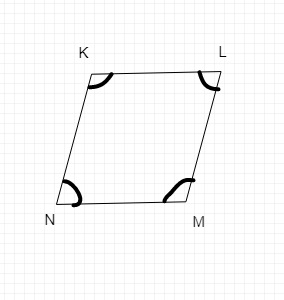# Draw a rough sketch of a quadrilateral KLMN. State,(a) two pairs of opposite sides,(b) two pairs of opposite angles,(c) two pairs of adjacent sides,(d) two pairs of adjacent angles.In the above figure,

(a) KL, NM, and KN, LM are pairs of opposite sides

(b) KLM and KNM; NML and NKL are pairs of opposite angles.

(c) KN and NM; KL and LM are two pairs of adjacent sides.

(d) KLM and NML; KNM and NKL are two pairs of adjacent angles.

Updated on: 10-Oct-2022

31 Views